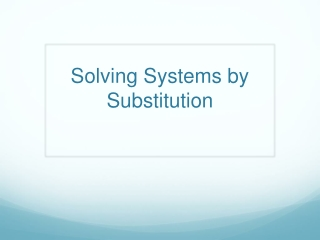DownloadDownload PresentationSolving Systems by Substitution

# Solving Systems by Substitution

Download Presentation## Solving Systems by Substitution

- - - - - - - - - - - - - - - - - - - - - - - - - - - E N D - - - - - - - - - - - - - - - - - - - - - - - - - - -
##### Presentation Transcript

1. Solving Systems by Substitution

2. Warm Up • Solve the following system by graphing:

3. Objective • The student will be able to solve systems of equations by substitution.

4. Exploration • Solving Systems of Equations by Substitution Exploration

5. Solving Systems by Substitution • You can solve systems of equations by substituting one equation into the other. • For example: • To solve this type of system you can simply set them equal to each other. See how y equals both 3 and 2x+1?

6. Try with a Partner… • Solve the following system by substitution:

7. Try With a Partner… • Solve the following system by substitution:

8. Substitution Continued… • Sometimes, systems are not always solved in slope intercept form… • Example: See how you can sub in first equation for y in the second? Now we have x we can solve for y.

9. Try with a Partner… • Solve the following system by substitution:

10. Try With a Partner… • Solve the following system by substitution:

11. On your own… • Solve the following system by substitution:

12. On your own… • Solve the following system by substitution:

13. Homework and Exit Ticket • Exit Ticket: • Solve the following: • Homework: • Worksheet